Assignment problem in linear programming : introduction and assignment model.Assignment problem is a special type of linear programming problem which deals with the allocation of the various resources to the various activities on one to one basis. It does it in such a way that the cost or time involved in the process is minimum and profit or sale is maximum. Though there problems can be solved by simplex method or by transportation method but assignment model gives a simpler approach for these problems.

In a factory, a supervisor may have six workers available and six jobs to fire. He will have to take decision regarding which job should be given to which worker. Problem forms one to one basis. This is an assignment problem.## 1. Assignment Model :

Suppose there are n facilitates and n jobs it is clear that in this case, there will be n assignments. Each facility or say worker can perform each job, one at a time. But there should be certain procedure by which assignment should be made so that the profit is maximized or the cost or time is minimized.In the table, Co ij is defined as the cost when j th job is assigned to i th worker. It maybe noted here that this is a special case of transportation problem when the number of rows is equal to number of columns.

## Mathematical Formulation:

Any basic feasible solution of an Assignment problem consists (2n – 1) variables of which the (n – 1) variables are zero, n is number of jobs or number of facilities. Due to this high degeneracy, if we solve the problem by usual transportation method, it will be a complex and time consuming work. Thus a separate technique is derived for it. Before going to the absolute method it is very important to formulate the problem.

Suppose x jj is a variable which is defined as

1 if the i th job is assigned to j th machine or facility

0 if the i th job is not assigned to j th machine or facility.

Now as the problem forms one to one basis or one job is to be assigned to one facility or machine.The total assignment cost will be given byThe above definition can be developed into mathematical model as follows:

Determine x ij > 0 (i, j = 1,2, 3…n) in order toSubjected to constraintsand x ij is either zero or one.

## Method to solve Problem (Hungarian Technique):

Consider the objective function of minimization type. Following steps are involved in solving this Assignment problem,

1. Locate the smallest cost element in each row of the given cost table starting with the first row. Now, this smallest element is subtracted form each element of that row. So, we will be getting at least one zero in each row of this new table.

2. Having constructed the table (as by step-1) take the columns of the table. Starting from first column locate the smallest cost element in each column. Now subtract this smallest element from each element of that column. Having performed the step 1 and step 2, we will be getting at least one zero in each column in the reduced cost table.

3. Now, the assignments are made for the reduced table in following manner.

(i) Rows are examined successively, until the row with exactly single (one) zero is found. Assignment is made to this single zero by putting square □ around it and in the corresponding column, all other zeros are crossed out (x) because these will not be used to make any other assignment in this column. Step is conducted for each row.

(ii) Step 3 (i) in now performed on the columns as follow:- columns are examined successively till a column with exactly one zero is found. Now , assignment is made to this single zero by putting the square around it and at the same time, all other zeros in the corresponding rows are crossed out (x) step is conducted for each column.

(iii) Step 3, (i) and 3 (ii) are repeated till all the zeros are either marked or crossed out. Now, if the number of marked zeros or the assignments made are equal to number of rows or columns, optimum solution has been achieved. There will be exactly single assignment in each or columns without any assignment. In this case, we will go to step 4.

4. At this stage, draw the minimum number of lines (horizontal and vertical) necessary to cover all zeros in the matrix obtained in step 3, Following procedure is adopted:

(iii) Now tick mark all the rows that are not already marked and that have assignment in the marked columns.

(iv) All the steps i.e. (4(i), 4(ii), 4(iii) are repeated until no more rows or columns can be marked.

(v) Now draw straight lines which pass through all the un marked rows and marked columns. It can also be noticed that in an n x n matrix, always less than ‘n’ lines will cover all the zeros if there is no solution among them.

5. In step 4, if the number of lines drawn are equal to n or the number of rows, then it is the optimum solution if not, then go to step 6.

6. Select the smallest element among all the uncovered elements. Now, this element is subtracted from all the uncovered elements and added to the element which lies at the intersection of two lines. This is the matrix for fresh assignments.

7. Repeat the procedure from step (3) until the number of assignments becomes equal to the number of rows or number of columns.

## Related Articles:

• Two Phase Methods of Problem Solving in Linear Programming: First and Second Phase
• Linear Programming: Applications, Definitions and Problems## OPERATIONS RESEARCH

Lesson 8. introduction and mathematical formulation.

Current course

## Operations Research - Definition and formulation of Assignment Problem | 12th Business Maths and Statistics : Chapter 10 : Operations Research

Chapter: 12th business maths and statistics : chapter 10 : operations research, definition and formulation of assignment problem.

Definition and formulation

Consider the problem of assigning n jobs to n machines (one job to one machine). Let C ij be the cost of assigning i th job to the j th machine and x ij represents the assignment of i th job to the j th machine.x ij is missing in any cell means that no assignment is made between the pair of job and machine.( i.e ) x ij = 0.

x ij presents in any cell means that an assignment is made their.In such cases x ij = 1

The assignment model can be written in LPP as followsSubject to the constrainsThe optimum assignment schedule remains unaltered if we add or subtract a constant from all the elements of the row or column of the assignment cost matrix.

If for an assignment problem all C ij > 0 then an assignment schedule (x ij ) which satisfies ∑ C ij x ij   = 0 must be optimal.

Related TopicsInternational Symposium on Intelligent Manufacturing and Service Systems

IMSS 2023: Advances in Intelligent Manufacturing and Service System Informatics pp 675–682 Cite as

## Mathematical Models for the Reviewer Assignment Problem in Project Management and a Case Study

• Zeynep Rabia Hosgor 13 ,
• Elifnaz Ozbulak 13 ,
• Elif Melis Gecginci 13 &
• Zeynep Idil Erzurum Cicek 13
• Conference paper
• First Online: 02 October 2023

50 Accesses

Part of the Lecture Notes in Mechanical Engineering book series (LNME)

Project management is a critical process for every institution and/or organization. This process should be managed as best as possible in order to manage resources and time well and at the same time achieve successful results. One of the reasons that make project management difficult is the increase in project proposals with the increase in reading rates and incentives. The evaluation process of the project proposals includes the assignment of an expert who will evaluate the project. This stage is solved with reviewer assignment problems in the literature. When the literature is examined, it is seen that the general aim of reviewer assignment problems is to maximize the degree of reviewer-project match. This study, in addition to the literature, it is aimed to minimize the evaluation time of the reviewers’ project proposals and to ensure a balanced distribution among the reviewers. When we look at the results of the test problems for this study, which has two objectives, maximum matching degree and minimum evaluation time, it is seen that the objectives have been met. In this way, the assignment, which was made manually and caused a waste of time, was completed in a fair and reliable way.

• project management
• reviewer assignment problem
• mathematical model
• optimization

This is a preview of subscription content, access via your institution .

• Available as PDF
• Own it forever
• Available as EPUB and PDF
• Durable hardcover edition
• Dispatched in 3 to 5 business days
• Free shipping worldwide - see info

Tax calculation will be finalised at checkout

Purchases are for personal use only

Hartvigsen, D., Wei, J.C.: The conference paper-reviewer assignment problem. Decis. Sci. 30 (3), 865–876 (1999)

Xinlian, L., Watanabe, T.: Automatic paper-to-reviewer assignment, based on the matching degree of the reviewers. Procedia Comput. Sci. 22 , 633–642 (2013)

Liu, O., Wang, J., Ma, J., Sun, Y.: An intelligent decision support approach for reviewer assignment in R&D project selection. Comput. Ind. 76 , 1–10 (2015)

Pradhan, D.K., Chakrabot, J., Choudhary, P., Nandi, S.: An automated conflict of interest based greedy approach for conference paper assignment system. J. Informetrics 14 (2), 101022 (2020)

Kat, B.: An algorithm and a decision support system for the panelist assignment problem: the case of TUBITAK. J. Fac. Eng. Archit. Gazi Univ. 36 (1), 69–87 (2021)

## Acknowledgments

This study is supported by TUBITAK 2209-A - Research Project Support Programme for Undergraduate Students and Eskisehir Technical University, Scientific Research Projects Committee (22LOP392).

## Author information

Authors and affiliations.

Eskisehir Technical University/Industrial Engineering, Eskisehir, Turkey

Zeynep Rabia Hosgor, Elifnaz Ozbulak, Elif Melis Gecginci & Zeynep Idil Erzurum Cicek

You can also search for this author in PubMed   Google Scholar

## Corresponding author

Correspondence to Zeynep Rabia Hosgor .

## Editor information

Editors and affiliations.

Istanbul Medipol University, Istanbul, Türkiye

Department of Industrial Engineering, Sakarya University, Serdivan, Sakarya, Türkiye

Faculty of Applied Sciences, Sakarya University of Applied Sciences, Kaynarca, Sakarya, Türkiye

Caner Erden

## Rights and permissions

Reprints and Permissions

© 2024 The Author(s), under exclusive license to Springer Nature Singapore Pte Ltd.

Cite this paper.

Hosgor, Z.R., Ozbulak, E., Gecginci, E.M., Cicek, Z.I.E. (2024). Mathematical Models for the Reviewer Assignment Problem in Project Management and a Case Study. In: Şen, Z., Uygun, Ö., Erden, C. (eds) Advances in Intelligent Manufacturing and Service System Informatics. IMSS 2023. Lecture Notes in Mechanical Engineering. Springer, Singapore. https://doi.org/10.1007/978-981-99-6062-0_63

DOI : https://doi.org/10.1007/978-981-99-6062-0_63

Published : 02 October 2023

Publisher Name : Springer, Singapore

Print ISBN : 978-981-99-6061-3

Online ISBN : 978-981-99-6062-0

eBook Packages : Engineering Engineering (R0)

Anyone you share the following link with will be able to read this content:

Provided by the Springer Nature SharedIt content-sharing initiative

• Find a journal
• Publish with us

## Assignment Model | Linear Programming Problem (LPP) | Introduction

What is assignment model.

→ Assignment model is a special application of Linear Programming Problem (LPP) , in which the main objective is to assign the work or task to a group of individuals such that;

i) There is only one assignment.

ii) All the assignments should be done in such a way that the overall cost is minimized (or profit is maximized, incase of maximization).

→ In assignment problem, the cost of performing each task by each individual is known. → It is desired to find out the best assignments, such that overall cost of assigning the work is minimized.

## For example:

Suppose there are 'n' tasks, which are required to be performed using 'n' resources.

The cost of performing each task by each resource is also known (shown in cells of matrix)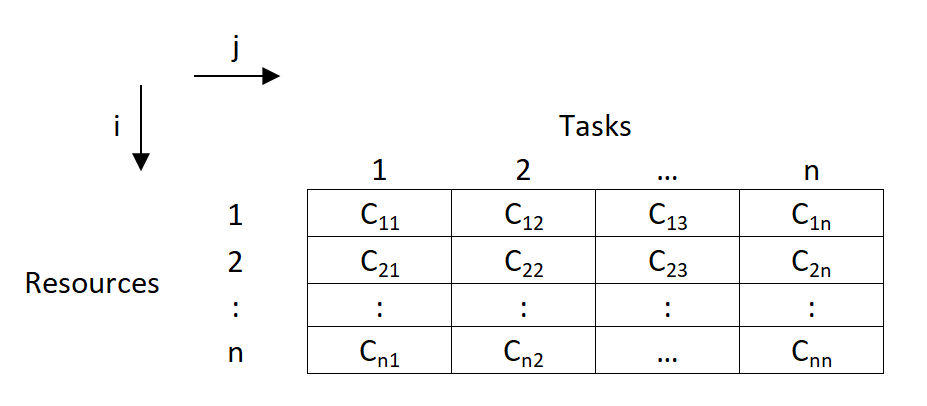• In the above asignment problem, we have to provide assignments such that there is one to one assignments and the overall cost is minimized.

## How Assignment Problem is related to LPP? OR Write mathematical formulation of Assignment Model.

→ Assignment Model is a special application of Linear Programming (LP).

→ The mathematical formulation for Assignment Model is given below:

→ Let, C i j \text {C}_{ij} C ij ​ denotes the cost of resources 'i' to the task 'j' ; such that→ Now assignment problems are of the Minimization type. So, our objective function is to minimize the overall cost.

→ Subjected to constraint;

(i) For all j t h j^{th} j t h task, only one i t h i^{th} i t h resource is possible:

(ii) For all i t h i^{th} i t h resource, there is only one j t h j^{th} j t h task possible;

(iii) x i j x_{ij} x ij ​ is '0' or '1'.

## Types of Assignment Problem:

(i) balanced assignment problem.

• It consist of a suqare matrix (n x n).
• Number of rows = Number of columns

## (ii) Unbalanced Assignment Problem

• It consist of a Non-square matrix.
• Number of rows ≠ \not=  = Number of columns

## Methods to solve Assignment Model:

(i) integer programming method:.

In assignment problem, either allocation is done to the cell or not.

So this can be formulated using 0 or 1 integer.

While using this method, we will have n x n decision varables, and n+n equalities.

So even for 4 x 4 matrix problem, it will have 16 decision variables and 8 equalities.

So this method becomes very lengthy and difficult to solve.## (ii) Transportation Methods:

As assignment problem is a special case of transportation problem, it can also be solved using transportation methods.

In transportation methods ( NWCM , LCM & VAM), the total number of allocations will be (m+n-1) and the solution is known as non-degenerated. (For eg: for 3 x 3 matrix, there will be 3+3-1 = 5 allocations)

But, here in assignment problems, the matrix is a square matrix (m=n).

So total allocations should be (n+n-1), i.e. for 3 x 3 matrix, it should be (3+3-1) = 5

But, we know that in 3 x 3 assignment problem, maximum possible possible assignments are 3 only.

So, if are we will use transportation methods, then the solution will be degenerated as it does not satisfy the condition of (m+n-1) allocations.

So, the method becomes lengthy and time consuming.

## (iii) Enumeration Method:

It is a simple trail and error type method.

Consider a 3 x 3 assignment problem. Here the assignments are done randomly and the total cost is found out.

For 3 x 3 matrix, the total possible trails are 3! So total 3! = 3 x 2 x 1 = 6 trails are possible.

The assignments which gives minimum cost is selected as optimal solution.

But, such trail and error becomes very difficult and lengthy.

If there are more number of rows and columns, ( For eg: For 6 x 6 matrix, there will be 6! trails. So 6! = 6 x 5 x 4 x 3 x 2 x 1 = 720 trails possible) then such methods can't be applied for solving assignments problems.

## (iv) Hungarian Method:

It was developed by two mathematicians of Hungary. So, it is known as Hungarian Method.

It is also know as Reduced matrix method or Flood's technique.

There are two main conditions for applying Hungarian Method:

(1) Square Matrix (n x n). (2) Problem should be of minimization type.

## Suggested Notes: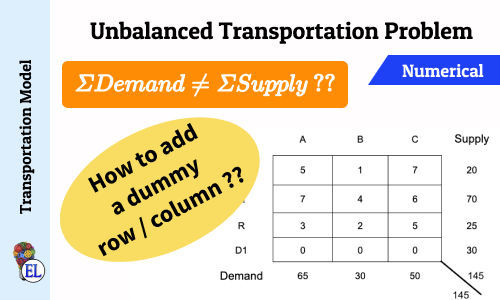## Unbalanced Transportation Problem Numerical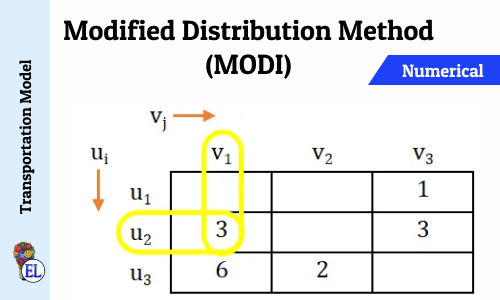## Modified Distribution Method (MODI) | Transportation Problem | Transportation Model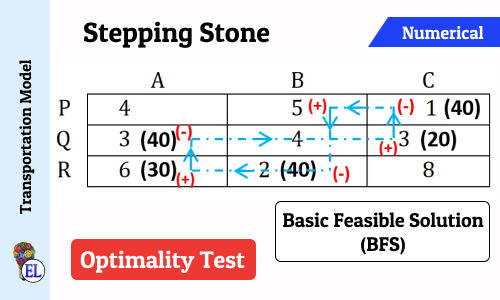## Stepping Stone | Transportation Problem | Transportation Model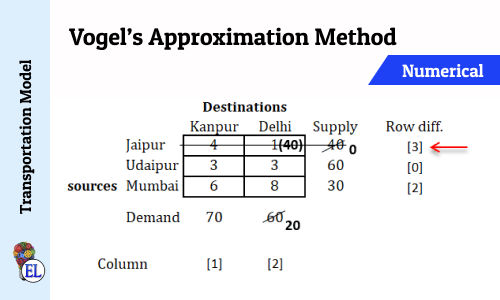## Vogel’s Approximation Method (VAM) | Method to Solve Transportation Problem | Transportation Model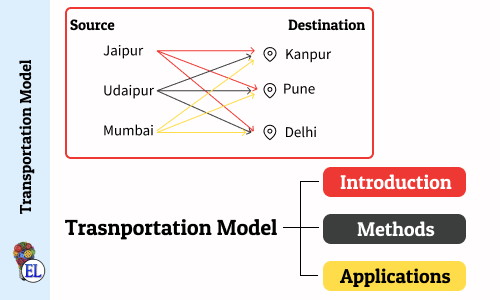## Transportation Model - Introduction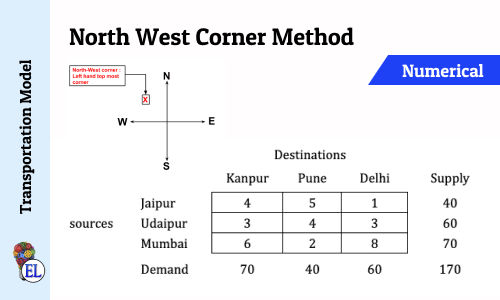## North West Corner Method | Method to Solve Transportation Problem | Transportation Model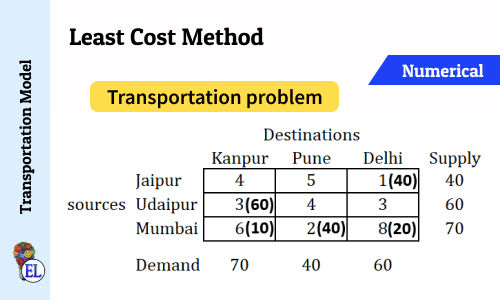## Least Cost Method | Method to Solve Transportation Problem | Transportation Model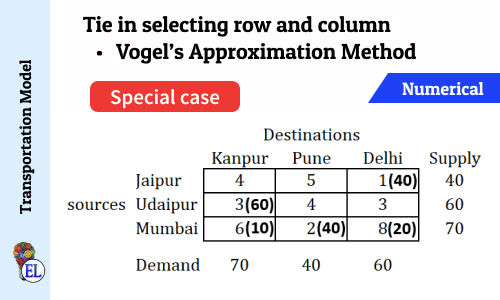## Tie in selecting row and column (Vogel's Approximation Method - VAM) | Numerical | Solving Transportation Problem | Transportation Model## Critical Path Method [CPM] - Steps and Introduction | Network Analysis | Operation Research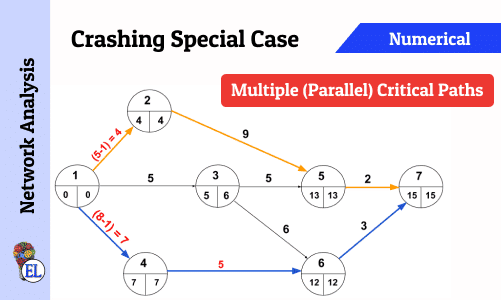## Crashing Special Case - Multiple (Parallel) Critical Paths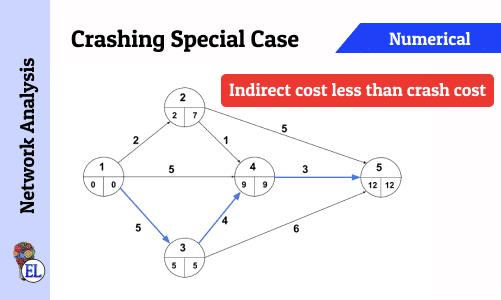## Crashing Special Case - Indirect cost less than Crash Cost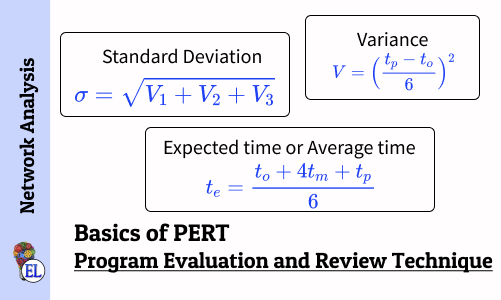## Basics of Program Evaluation and Review Technique (PERT)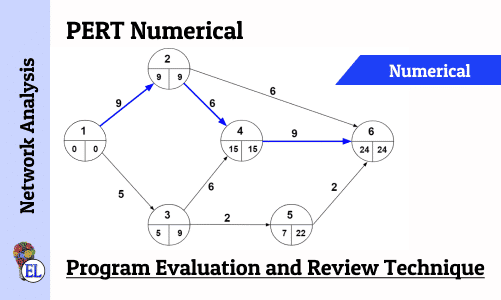## Numerical on PERT (Program Evaluation and Review Technique)## Network Analysis - Dealing with Network Construction Basics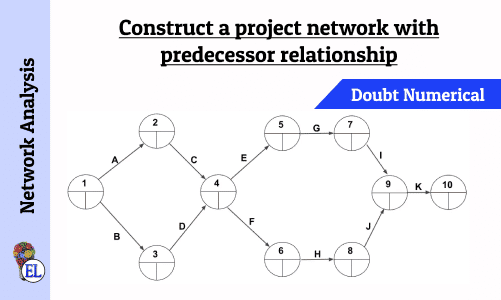## Construct a project network with predecessor relationship | Operation Research | Numerical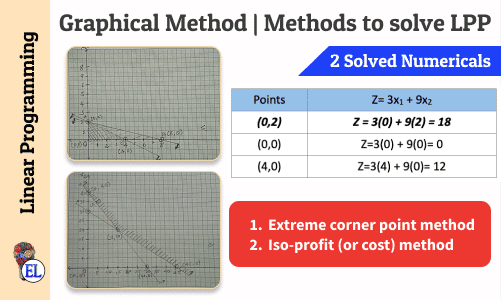## Graphical Method | Methods to solve LPP | Linear Programming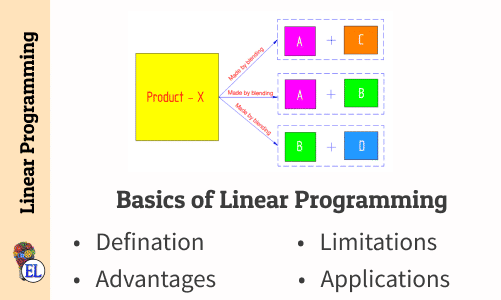## Basics of Linear Programming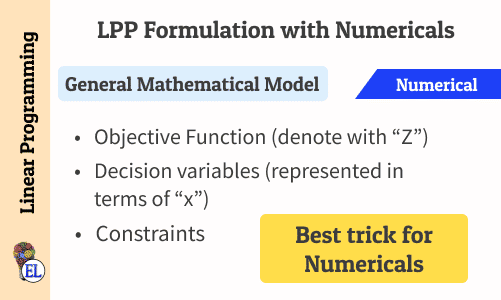## Linear Programming Problem (LPP) Formulation with Numericals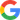All comments that you add will await moderation. We'll publish all comments that are topic related, and adhere to our Code of Conduct .

## Post comment## Education Lessons

Stay in touch, [notes] operation research, [notes] dynamics of machinery, [notes] maths, [notes] science, [notes] computer aided design.#### IMAGES

1. Assignment Problem2. [Solved] Mathematical modeling Question 4.  Deﬁne the function f3. Assignment problems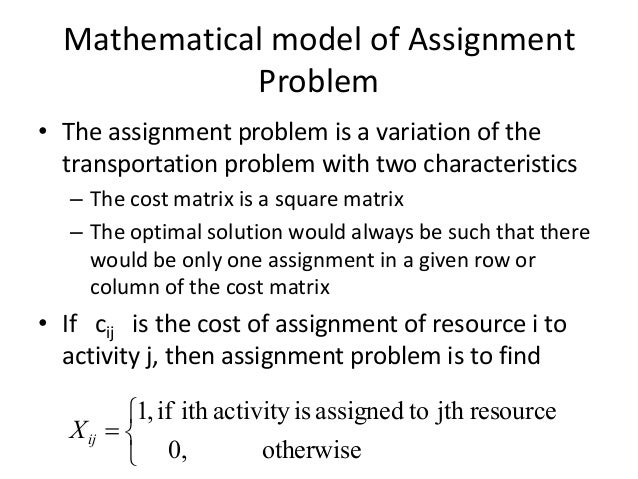4. PPT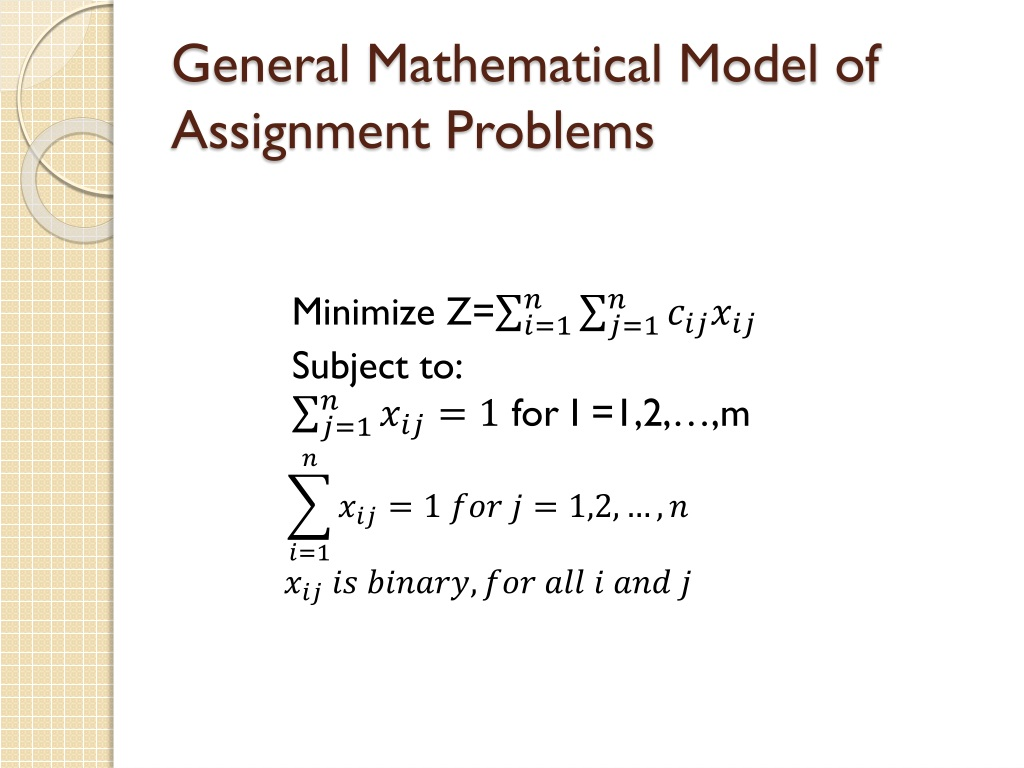5. MATHEMATICAL FORMULATION OF ASSIGNMENT PROBLEM BY DR KUNAL KHATRI #STATISTICS4ALL #ASSIGNMENT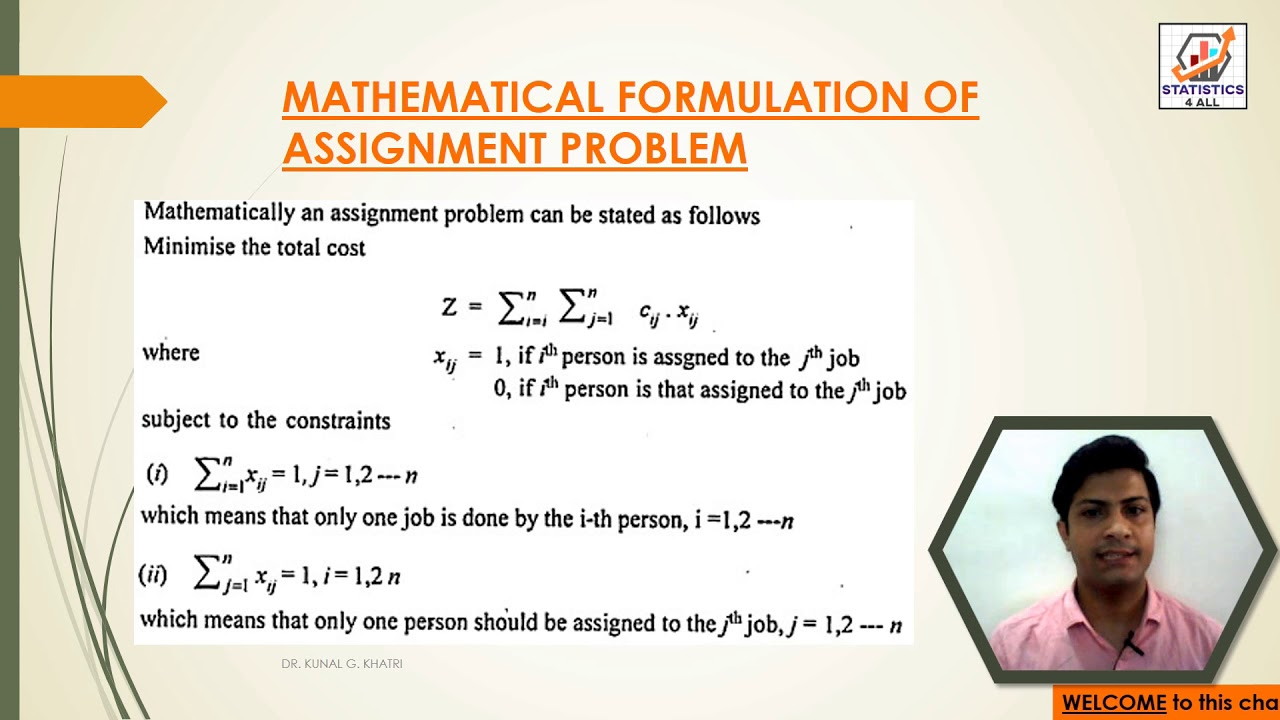6. Assignment problem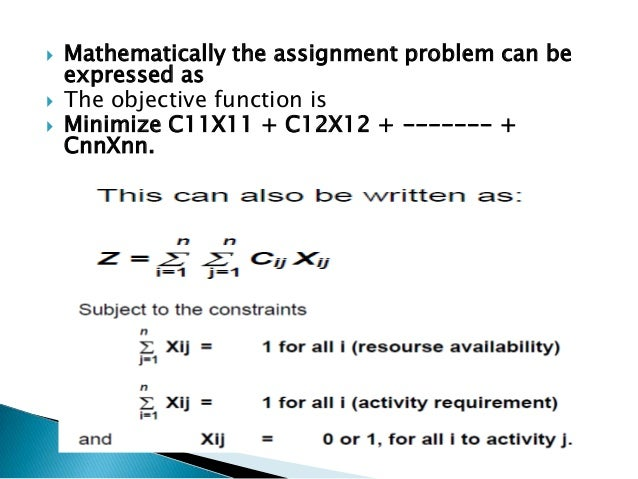#### VIDEO

1. Lecture 4: Different types of Mathematical Models

2. Assignment work of mathematical method of bsc math3rdsem,#ddugkp #laplacetransform#2023-24 #trending

3. Mathematical formulation of Assignment problem

4. AF3 mathematical model team 4 group 53

5. Revised question in Assignment work of mathematical method of bsc3rd sem#assignmentwork #ddu

6. General Mathematical Model

Math assignments can be quite challenging, especially if you don’t have a good understanding of the concepts involved. When you find yourself struggling with your math assignments, it is normal to feel stressed out and overwhelmed.

2. What Does “sum” Mean in Math?

In mathematics, adding numbers, items or amounts produces a sum. The word also refers to a group of arithmetic problems given as a classroom assignment. As a verb, to sum is to find the total of added amounts.

3. Expert Tips for Choosing the Right Company to Do Your Math Assignment

Mathematics is a subject that requires a lot of skills and attention. When you are faced with a math assignment, it can be challenging, especially if you have other commitments or struggle with the subject. Fortunately, you do not have to s...

4. Assignment Problem in Linear Programming : Introduction and

Assignment problem is a special type of linear programming problem which deals with the allocation of the various resources to the various activities on one

5. ASSIGNMENT PROBLEM

LINEAR PROGRAMMING PROBLEM. 13 / 62. Page 140. GRAPHICAL SOLUTION OF LPP MODELS. If the

6. Modeling and Solution for Assignment Problem

This is the mathematical model of the minimax assignment problem. It is an integer programming, where the objective function is nonlinear. There is no

7. Assignment Problem

This lecture discusses the assignment problems Other videos @DrHarishGarg Assignment Problem - Mathematical Models: Link:

8. Mathematical Formulation of the Problem

The assignment problem is a linear programming problem that entails allocating resources to individual tasks. It does so that the process's cost or time is kept

9. Assignment problem

A naive solution for the assignment problem is to check all the assignments and calculate the cost of each one. This may be very inefficient since, with n

10. Assignment Problem

General form of an Assignment Problem: The assignment problem can be stated in the form of n × n, matrix [cij] called the cost of effectiveness matrix, where

11. Lesson 8. INTRODUCTION AND MATHEMATICAL FORMULATION

The assignment problem deals in allocating the various origins (jobs) to equal number of destinations (persons) on a one to one basis in such a way that the

12. Definition and formulation of Assignment Problem

(i.e) xij = 0. xij presents in any cell means that an assignment is made their.In such cases x

13. Mathematical Models for the Reviewer Assignment Problem in

Project management is a critical process for every institution and/or organization. This process should be managed as best as possible in

14. Assignment Model

mathematical formulation of Assignment Model. → Assignment Model is a